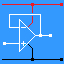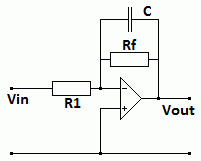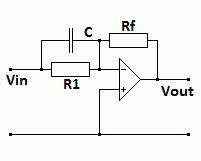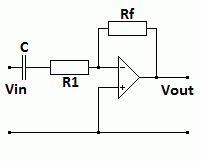RANDOM PAGE

SITE SEARCH

LOG
IN

HELP

# Active Filters

This is the AQA version closing after June 2019. Visit the the version for Eduqas instead.

## Active Filters

Inverting Op-Amp First Order Active Filters

• These filters add a capacitor to the usual inverting amplifier circuit.
• The capacitor is a frequency dependent component so the amplifier gain now depends on the frequency.

### Low Pass - Treble Cut• For DC and low frequencies, the capacitor has no effect.
• The DC and low frequency gain = -Rf / R1.
• Well above the breakpoint frequency, the capacitor
reactance is very low.
• At high frequencies, the gain drops to zero due
to the negative feedback through the capacitor.
• The input resistance equals R1.

### Treble Boost• At low frequencies, the capacitor has no effect.
• At low frequencies, the gain = -Rf / R1.
• At high frequencies the capacitor reduces R1.
• At high frequencies the gain increases.

### High Pass - Bass Cut• The capacitor blocks DC and low frequencies.
• The capacitor passes high frequencies easily.
• The amplifier provides gain.
• DC and low frequencies the gain = 0.
• Well above the breakpoint frequency, the gain = -Rf / R1.

### Bass Boost• For DC and low frequencies, the capacitor has no effect.
• Rf must be large.
• At low frequencies the gain is large = -Rf / R1.
• At high frequencies, Xc is low so the gain drops to zero.
• The input resistance equals R1

### Breakpoint Frequency Calculations

• These calculations apply to both passive and active filters.
• At the breakpoint frequency, the reactance of the capacitor equals the resistance of the resistor.

• R = Xc

• Xc = 1 / (2 π f C)

• R = 1 / (2 π f C)

• R f = 1 / (2 π C)

• f = 1 / (2 π R C)

• C = 1 / (2 π R f)

reviseOmatic V3     Contacts, ©, Cookies, Data Protection and Disclaimers Hosted at linode.com, London